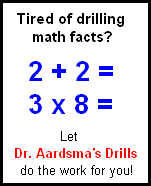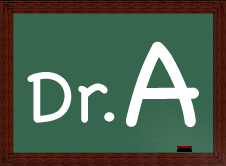Home
Convert 30-Day Trial to Full Registration
Change My Student's Lesson Number
Change the Question Skipping Behavior of My Student's Checker
Choose Problems to be Included in My Students' Lesson
Reset My Student's Current LessonTips for New Users
Use of Calculators in Saxon MathHow to Prepare for ACT/SAT
The Creator of Saxon MathUse of Calculators for Saxon Math

Should students be allowed to use a calculator for Saxon Math?

Saxon makes its own recommendation on this question. At the beginning of the Saxon Math student textbooks you will find a "List of Materials" needed for the course. If it lists "scientific calculator" or "calculator with memory functions", then your student should be allowed to use the designated calculator. (Note that there are two kinds of calculators that students can use for math: a calculator with memory functions [for the lower grades] and a scientific calculator with math and science functions [for the higher grades].)

Before Saxon Math 7/6, no calculators should be used. Saxon Math 7/6 allows the use of a calculator with memory functions. All Saxon Math after 7/6 allows the use of a scientific calculator. Dr. Aardsma supports these recommendations.Mental Math

In some grades Saxon Math has a "Mental Math" section with each lesson. The idea is that the teacher should read aloud the mental math problems to the student and the student should perform the calculations mentally (no pencil and paper or calculator). Mental math ability pays lifelong benefits and improves markedly with practice. Many students can perform mental math much faster than using a calculator, and this skill is especially important for students heading into a field that relies heavily on math.

Math Drills

Additionally, students should be doing daily math drills from 1st grade through 6th grade (see Dr. Aardsma's Math Drill). No calculators should be used for these drills. It is mandatory that students memorize the basic math facts as a foundation to all further work in math.